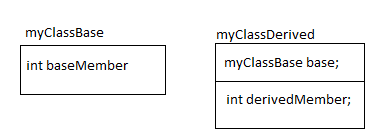# Inheritance

Lets consider these two classes -
```class myClassBase
{
public:
int baseMember;
myClassBase() { baseMember = 0; }
int baseMethod(int x) { return baseMember + x; }
};
class myClassDerived : public myClassBase
{
public:
int derivedMember;
myClassDerived() { derivedMember = 0; }
int derivedMethod(int x) { return derivedMember + x; }
};
```

The equivalent C code will be
```struct myClassBase
{
int baseMember;
};
struct myClassDerived
{
struct myClassBase base;
int derivedMember;
};
void myClassBase_ctor(struct myClassBase *this)
{
this->baseMember = 0;
}
int myClassBase_baseMethod(struct myClassBase *this, int x)
{
return this->baseMember + x;
}
void myClassDerived_ctor(struct myClassDerived *this)
{
myClassBase_ctor(this);
this->derivedMember = 0;
}
int myClassDerived_derivedMethod(struct myClassDerived *this, int x)
{
return this->derivedMember + x;
}
```

The relation between the classes can be described by a diagram as:Here are few points to understand this:
• The first member of the derived structure is of the type base.
• If the object of the derived is casted (using pointers) as the base member, it this will point to first member of the struct which is of type base struct.
• Defining the structure this way helps in implementing the polymorphism (the pointer of derived can used as pointer of base).
• The constructor of the derived class first calls the base class constructor. This ensures that the base class is constructed first then the derived class.
• The constructor of the derived class can specify which constructor of the base class should be called. This is C++ language struct. If nothing is specified, the default constructor(with no parameter) is called.
• Constructor of derived class is called from the code where the object is instantiated. The constructor of of derived class calls the constructor of its base.